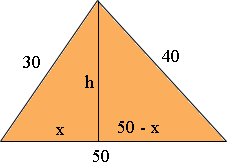SEARCH HOMEMath Central Quandaries & QueriesSubject: Math Name: Sharon Who are you: Parent I am needing to determine the height of a triangle with a 50 ft base and one side is 40 ft the other side being 30 ft.In the diagram below h is the height of the triangle in feet.By Pythagoras' Theorem

x2 + h2 = 302

and

(50 - x)2 + h2 = 402

Subtracting the first equation from the second gives

(50 - x)2 - x 2 = 402 - 302

Solve this equation for x and substitute this value into the first equation and solve for h.

PennyMath Central is supported by the University of Regina and The Pacific Institute for the Mathematical Sciences.